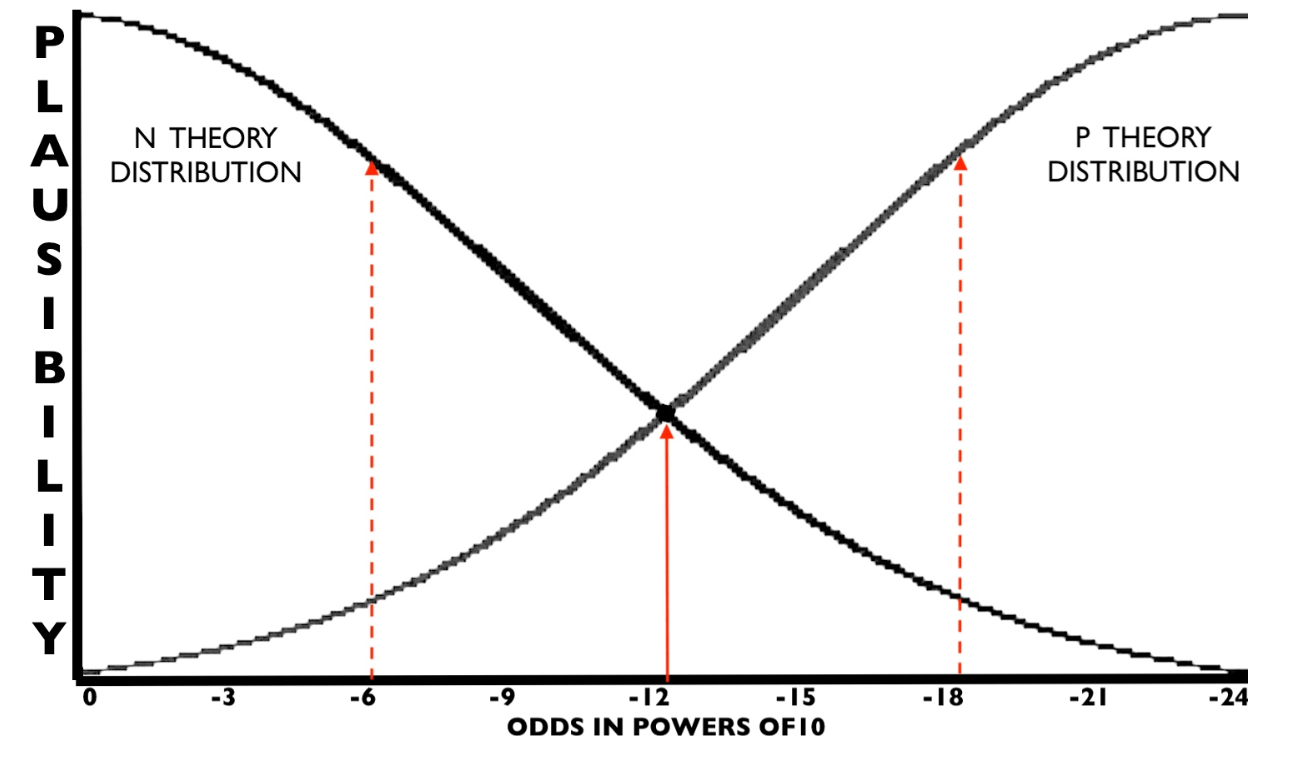By the Principle of the Long Run, a coincidence is always deemed to be a chance event, no matter how long the odds. If paranormal coincidences exist, the normal scientific approach means that they would be mixed in with chance events and made non-significant.

Science rules out of existence the very phenomenon that it should be investigating: that some coincidences may be caused by an undefined non-chance process, X.

This assumption that coincidences must always be created by chance means that the conventional criterion for statistical significance does not work for coincidences. Null hypothesis significance testing (NHST) is not applied.

Thus P < .000000000001 is Not Significant, according to the accepted scientific view

The standard criterion for rejecting a null hypothesis H0 is p<.05 or p<.01. For coincidences, however, this probability can be as low as p<.000000000001 (10-12) or even much lower and the occurrence is accepted as falling within chance levels.

This double standard in evaluating the statistical significance of coincidences is interesting: no matter how improbable the outcome, the event is said to be consistent with the null hypothesis.

How can we escape this paradox? I wish to suggest a hypothesis that my totally skeptical friends and readers will find apocryphal. To avoid miscounting paranormal events as chance events, and defining them out of existence, it is necessary to hypothesise two populations of coincidental events, those due purely to chance, and those that have a putative paranormal source.

Imagine a coincidence with an odds of 10 to the minus 12. This is one chance in a million million. Consider the  possibility that there is an alternative to the normal, null hypothesis, which we can call the ‘paranormal hypothesis’:

H0: The null hypothesis – what occurred was purely chance

H1: The paranormal hypothesis – what occurred was too improbable to be caused by chancePlausibility of events with different odds with a duplex of distributions for normal (chance) and paranormal (non-chance) coincidences. The shape of the distributions is normal and, speculatively, the dispersions are set to provide a cross-over point with odds of 10-12  where the Bayes factor is one (thin vertical line). Events with odds to the left of this line have Bayes Factors < 1.0 and to the right of this line, Bayes Factor > 1.0. The two dotted lines indicate events with odds of 10-6 and 10-18 where the Bayes factor is .20 and 5.0 respectively.

According to this hypothetical framework, a cross-over point with a Bayes Factor of 1.0 occurs with an odds value of 10-12. In order to specify the form of these distributions it would be necessary to collect large numbers of data points with exact odds values.The hypothesised existence of a separate population of paranormal events places N Theory and P Theory on an equal footing as explanations and avoids a double standard.

Is the theory correct?  It is impossible to say.Author

## 2 thoughts on “The Paradox of Coincidence”

1.cindy knoke says:

“If paranormal coincidences exist, the normal scientific approach means that they would be mixed in with chance events and made invisible.”
Not invisible, but statistically ‘insignificant.’
I am married to a PhD biostatistician.
Statisical inisiginifance is the launching for phenomena that is the most provactive and interesting.

1.dfmarks says:

Thank you for your interesting comment. The person who experiences an anomalous event will remember it forever. It may even have life changing consequences. The biostatistician will be more inclined to believe with R A Fisher that: “The million, million, million… to one chance happens once in a million, million, million… times no matter how surprised we may be that it results in us.”